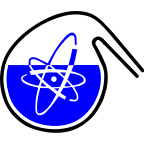#RadChem 2022

May 15 – 20, 2022
Casino Conference Centre
Europe/Prague timezone

## Trivalent Actinide Ions Showing Tenfold Coordination in Solution

May 16, 2022, 2:20 PM
20m
Marble Hall

### Marble Hall

Verbal Chemistry of Actinide and Trans-actinide Elements

### Speaker

Dr Patrik Weßling (Heidelberg University)

### Description

Trivalent actinides generally exhibit ninefold coordination in solution. nPr-BTP, a tridentate nitrogen donor ligand, is known to form ninefold coordinated 1:3 complexes, [An(nPr-BTP)$_3$]$^{3+}$ (An = U, Pu, Am, Cm) in solution.
We report the first Cm(III) complex with tenfold coordination in solution [Cm(nPr-BTP)$_3$(NO$_3$)]$^{2+}$. This species was identified using time-resolved laser fluorescence spectroscopy (TRLFS), vibronic side band spectroscopy (VSBS), X-ray photoelectron spectroscopy (XPS) and density functional theory (DFT).
Adding nitrate to a solution of the [Cm(nPr-BTP)$_3$]$^{3+}$ complex in 2-propanol shifts the Cm(III) emission band from 613.1 nm to 617.3 nm. This bathochromic shift is due to a higher coordination number of the Cm(III) ion in solution, in agreement with the formation of the [Cm(nPr-BTP)$_3$(NO$_3$)]$^{2+}$ complex. No such shift is observed when adding other anions (NO$_2^{-}$, CN$^{-}$ and OTf$^{-}$).
The formation of the [Cm(nPr-BTP)$_3$(NO$_3$)]$^{2+}$ complex was studied as a function of NO$_3^{-}$ and nPr-BTP concentrations. Slope analyses confirm the addition of one nitrate anion to the [Cm(nPr-BTP)$_3$]$^{3+}$ complex. Experiments with varied nPr-BTP concentration show that [Cm(nPr-BTP)$_3$(NO$_3$)]$^{2+}$ only forms at nPr-BTP concentrations below 10$^{-4}$ mol/L. For concentrations greater than 10$^{-4}$ mol/L only [Cm(nPr-BTP)$_3$]$^{3+}$ is observed.
VSBS allows observing vibrations of coordinated functional groups. The vibronic side band of the [Cm(nPr-BTP)$_3$(NO$_3$)]$^{2+}$ species exhibits a nitrate stretching mode not observed in the [Cm(nPr-BTP)$_3$]$^{3+}$ complex. Moreover, XPS on [Am(nPr-BTP)$_3$(NO$_3$)]$^{2+}$ yields signals from both non-coordinated and coordinated nitrate. DFT calculations reveal that the energetically most favoured structure is obtained if the nitrate is positioned on the C$_2$ axis of the D$_3$ symmetrical [Cm(nPr-BTP)$_3$]$^{3+}$ complex with a bond distance of 413 pm.
Combining results from TRLFS, VSBS, XPS and DFT provides sound evidence for a unique tenfold coordinated Cm(III) complex in solution - a novelty in An(III) solution chemistry.

### Primary authors

Dr Patrik Weßling (Heidelberg University) Andreas Geist (Karlsruhe Institute of Technology (KIT)) Petra Panak (J.)

### Presentation materials

There are no materials yet.